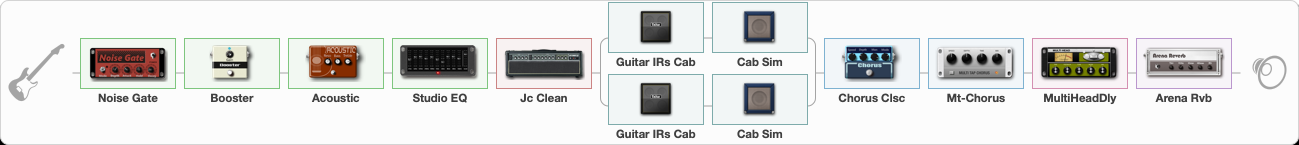# Metallica -Sanitarium

Discussion in 'ToneLib-GFX presets' started by davidtaylors, May 17, 2020.

1. ### davidtaylorsWell-Known Member

Metallica -Sanitarium

Preset name: Sanitarium

Neck pickup. Great for the clean tone.

Effects chain:Effect: "Noise Gate" (Dynamics / Filter), active - "yes"
{
"Mode" = Manual
"Depth" = 50
"Threshold" = 50
"Attack" = 142
"Hold" = 1202
"Decay" = 1836
}

Effect: "Booster" (Dynamics / Filter), active - "yes"
{
"Gain" = 54
}

Effect: "Acoustic" (Dynamics / Filter), active - "yes"
{
"Style" = Pop music
"Resonance" = 35
"Bass" = 55
"Treble" = 65
}

Effect: "Studio EQ" (Dynamics / Filter), active - "yes"
{
"31 Hz" = 9
"62 Hz" = 15
"125 Hz" = 8
"250 Hz" = -6
"500 Hz" = -9
"1 kHz" = 3
"2 kHz" = 6
"4 kHz" = 7
"8 kHz" = 7
"16 kHz" = 8
"Level (dB)" = -8
}

Effect: "Jc Clean" (Amp simulators), active - "yes"
{
"Gain" = 48
"Bass" = 54
"Middle" = 29
"Treble" = 69
"Presence" = 87
"Master" = 59
"Level (dB)" = -3
}

Effect: "Splitter" (Dynamics / Filter), active - "yes"
{
"A-Bypass" = Off
"A-Pan" = 0
"A-Level" = 55
"B-Bypass" = Off
"B-Pan" = 0
"B-Level" = 55

'A' branch:
{

Effect: "Guitar IRs Cab" (Cabinets), active - "yes"
{
"Model" = Engl Pro (4x12")
"Mic Position" = Edge
"Mic Distance" = Middle
"Low Cut (Hz)" = 60
"Hi Cut (kHz)" = 20.0
"Mix" = 100
"Level (dB)" = 0
}

Effect: "Cab Sim" (Cabinets), active - "yes"
{
"Model" = 1x12" US Delux
"Level (dB)" = 8
}
}
'B' branch:
{

Effect: "Guitar IRs Cab" (Cabinets), active - "yes"
{
"Model" = Vox AC30 (2x12")
"Mic Position" = Edge
"Mic Distance" = Middle
"Low Cut (Hz)" = 60
"Hi Cut (kHz)" = 20.0
"Mix" = 100
"Level (dB)" = 0
}

Effect: "Cab Sim" (Cabinets), active - "yes"
{
"Model" = 4x10" Tweed P10r
"Level (dB)" = 8
}
}
}

Effect: "Chorus Clsc" (Modulation / Sfx), active - "yes"
{
"Speed" = 4.7
"Depth" = 58
"Center" = 7.0
"Mode" = Stereo
}

Effect: "Mt-Chorus" (Modulation / Sfx), active - "yes"
{
"Speed" = 5.3
"Depth" = 92
"Time" = 2.5
"Mix" = 67
}

Effect: "MultiHeadDly" (Delay), active - "yes"
{
"Time" = 205
"Feedback" = 40
"Tone" = 58
"MODE" = Rhythm X-0-X-X
"Mix" = 71
}

Effect: "Arena Rvb" (Reverb), active - "yes"
{
"Time" = 7.3
"PreDelay" = 31
"LoDamp" = 18
"HiDamp" = 21
"Mix" = 91
}

Note: You will need to download and install the ToneLib-GFX software to use the preset.

File size:
1.3 KB
Views:
9,539